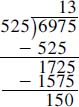NextPrevious

# What is Euler’s method?

Swiss mathematician Leonhard Euler (1707–1783) was one of the most prolific mathematicians who ever lived. He developed Euler’s method, which is a way of determining the largest divisor of two numbers. For example, if we want to find the largest divisor of the numbers 6,975 and 525, we consider one to be the large number and the other the small number. We already know both numbers are divisible by 0 and 5 (as they both end in 5), but how do we determine if they have a larger divisor? And if so, what is that number?

The key is to take the remainders of long division until we arrive at a remainder of zero. In this instance, we would first divide 6,975 by 525; the answer comes out to 13 and a remainder:Take the remainder—in this case, 150—and then divide it into the 525. Take that remainder (which turns out to be 75) and divide it into the 150 remainder. The next iteration leads to no remainder (zero).

 Large Number Small Number Remainder 6975 525 150 525 150 75 150 75 0

Thus, taking the number before we reached the zero—or 75—gives us the largest common divisor of both 6,975 and 525.

Close

This is a web preview of the "The Handy Math Answer Book" app. Many features only work on your mobile device. If you like what you see, we hope you will consider buying. Get the App